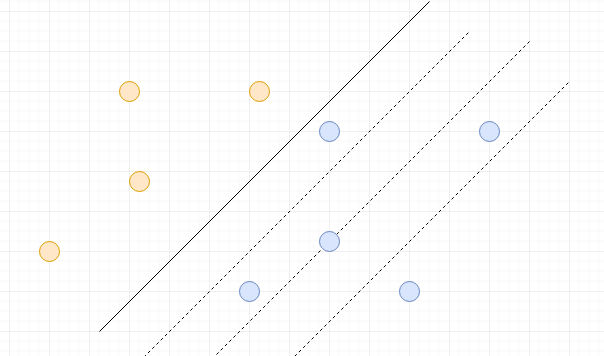Balanced Partition
Tag(s):

## Algorithms, Bubble sort algorithm, Easy-Medium, Rotation, Sorting, rotations

Problem
Editorial
Analytics

There are $n$ houses in the village Dholakpur. The location of each house in the village can be given as $(x_i, y_i)$ in the Cartesian coordinate plane. There are $h_i$ persons living in the $i^{th}$ house. Central electricity authority of the village is set to built a wire line across the village. The wire line is supposed to constructed in a way such that it is the north-east direction. In other words the wire line is parallel to the line $y = x$. Given that the construction of such line is considered to be effective only if the number of persons living in its left and right side are equal, can you tell if the construction of such wire line is possible?Note: If the wire line passes through any house, the house is not considered in either half.

Constraints:

• $1 \le t \le 100$
• $2 \le n \le 2 \times 10^3$
• $-10^3 \le x_i, y_i \le 10^3$
• $1 \le h_i \le 10^3$
• It is guaranteed that no two houses are at the same location.

Input Format:

The first line contains a single integer $t$  denoting the number of test cases.

The first line of each test case contains $n$  i.e the number houses in the village. Next $n$ lines contains $3$ space-separated integers $x_i, y_i \text{ and } h_i$

Output Format:

Print $t$  lines each containing a "YES" or "NO" (without quotes). $i^{th}$ line corresponds to the answer of the $i^{th}$ testcase.

SAMPLE INPUT
3
3
-1 1 3
-2 1 1
1 -1 4
3
1 1 2
-1 1 1
1 -1 2
4
0 2 3
-1 1 2
0 1 2
3 1 5

SAMPLE OUTPUT
YES
NO
YES

Explanation
• Testcase 1: There are infinitely many wire lines which divide in $2$ equal parts. Any line $y = x + c, -2 \lt c \lt 2$
• Testcase 2: No matter how hard you try, you will not get any such wire line.
• Testcase 3: Exactly $1$ line, i.e. $y = x + 1$ exists. Note that for this line the two persons living at $(0, 1)$ are neither considered on the left half, nor on the right.
Time Limit: 1.0 sec(s) for each input file.
Memory Limit: 256 MB
Source Limit: 1024 KB
Marking Scheme: Marks are awarded when all the testcases pass.
Allowed Languages: Bash, C, C++, C++14, Clojure, C#, D, Erlang, F#, Go, Groovy, Haskell, Java, Java 8, JavaScript(Rhino), JavaScript(Node.js), Julia, Kotlin, Lisp, Lisp (SBCL), Lua, Objective-C, OCaml, Octave, Pascal, Perl, PHP, Python, Python 3, R(RScript), Racket, Ruby, Rust, Scala, Swift, Swift-4.1, TypeScript, Visual Basic

## CODE EDITOR

Initializing Code Editor...

## This Problem was Asked inChallenge Name

June Easy '18

OTHER PROBLEMS OF THIS CHALLENGE
• Basic Programming > Implementation
• Math > Combinatorics
• Algorithms > Searching
• Math > Linear Algebra
• Algorithms > Graphs